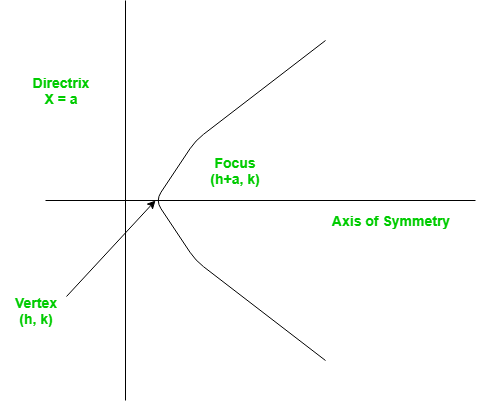Related Articles
Java Program for Finding the vertex, focus and directrix of a parabola
• Last Updated : 05 Dec, 2018

A set of points on a plain surface that forms a curve such that any point on that curve is equidistant from the focus is a parabola.
Vertex of a parabola is the coordinate from which it takes the sharpest turn whereas a is the straight line used to generate the curve.The standard form of a parabola equation is. Given the values of a, b and c; our task is to find the coordinates of vertex, focus and the equation of the directrix.

Example –

```Input : 5 3 2
Output : Vertex:(-0.3, 1.55)
Focus: (-0.3, 1.6)
Directrix: y=-198
Consult the formula below for explanation.
```

## Recommended: Please try your approach on {IDE} first, before moving on to the solution.

 `// Java program to calculate Vertex, Focus and Directrix`` ` `public` `class` `TriangularPyramidNumber {``    ``public` `static` `void` `parabola(``float` `a, ``float` `b, ``float` `c)``    ``{``        ``System.out.println(``"Vertex: ("` `+ (-b / (``2` `* a)) + ``", "``                           ``+ (((``4` `* a * c) - (b * b)) / (``4` `* a)) + ``")"``);`` ` `        ``System.out.println(``"Focus: ("` `+ (-b / (``2` `* a)) + ``", "``                           ``+ (((``4` `* a * c) - (b * b) + ``1``) / (``4` `* a)) + ``")"``);`` ` `        ``System.out.println(``"Directrix: y="``                           ``+ (``int``)(c - ((b * b) + ``1``) * ``4` `* a));``    ``}``    ``public` `static` `void` `main(String[] args)``    ``{``        ``float` `a = ``5``, b = ``3``, c = ``2``;``        ``parabola(a, b, c);``    ``}``}`` ` `// Contributed by _omg`
Output:
```Vertex: (-0.3, 1.55)
Focus: (-0.3, 1.6)
Directrix: y=-198
```

Please refer complete article on Finding the vertex, focus and directrix of a parabola for more details!

My Personal Notes arrow_drop_up